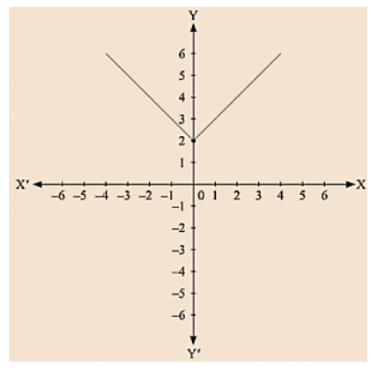# Draw the graph of y = |×|.

Question:

Draw the graph of y = |×|.

Solution:

We are given, y = |x|

Substituting x = 1, we get y = 1

Substituting x = - 1, we get y = 1

Substituting x = 2, we get y = 2

Substituting x = - 2, we get y = 2For every value of x, whether positive or negative, we get y as a positive number.